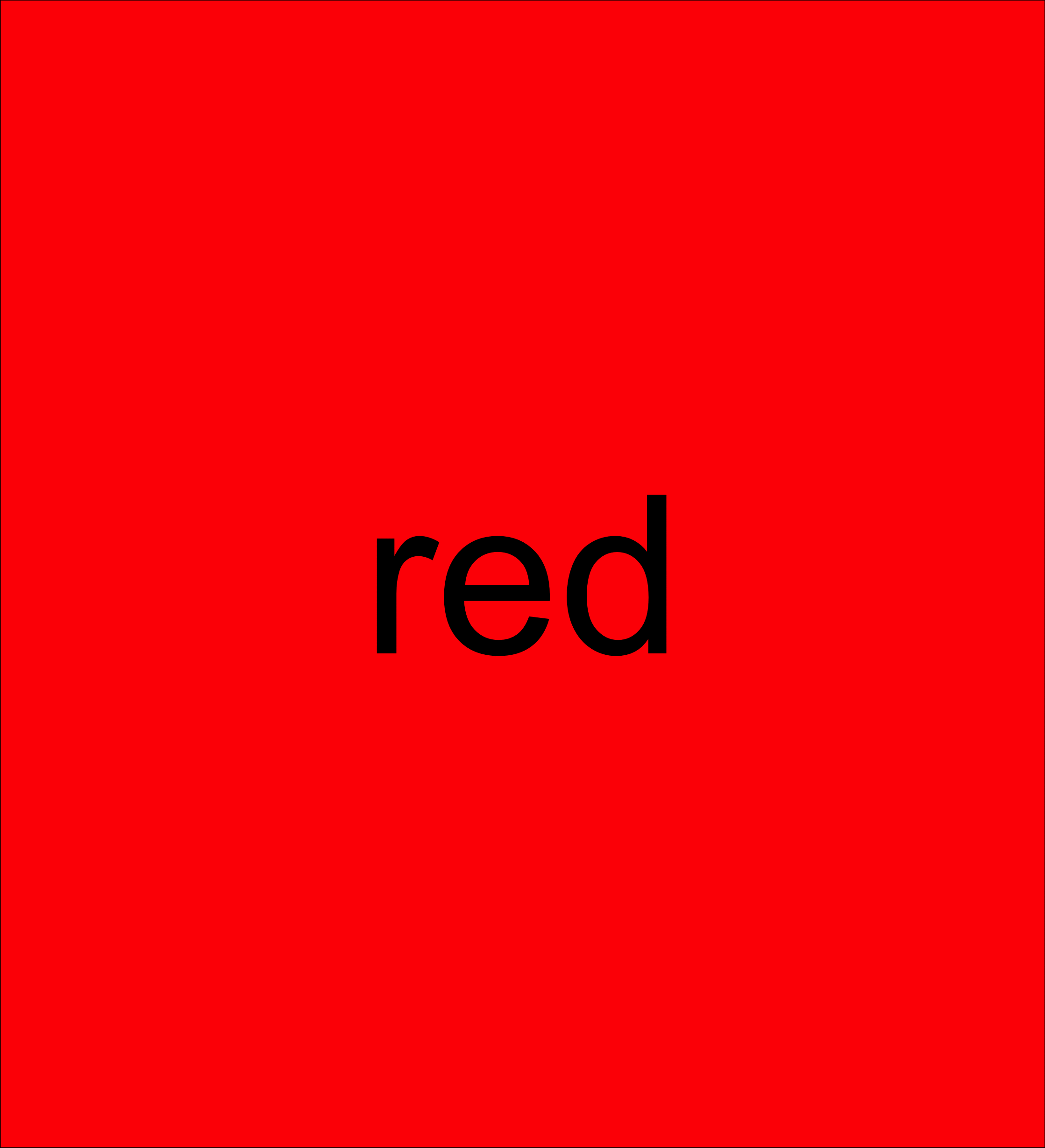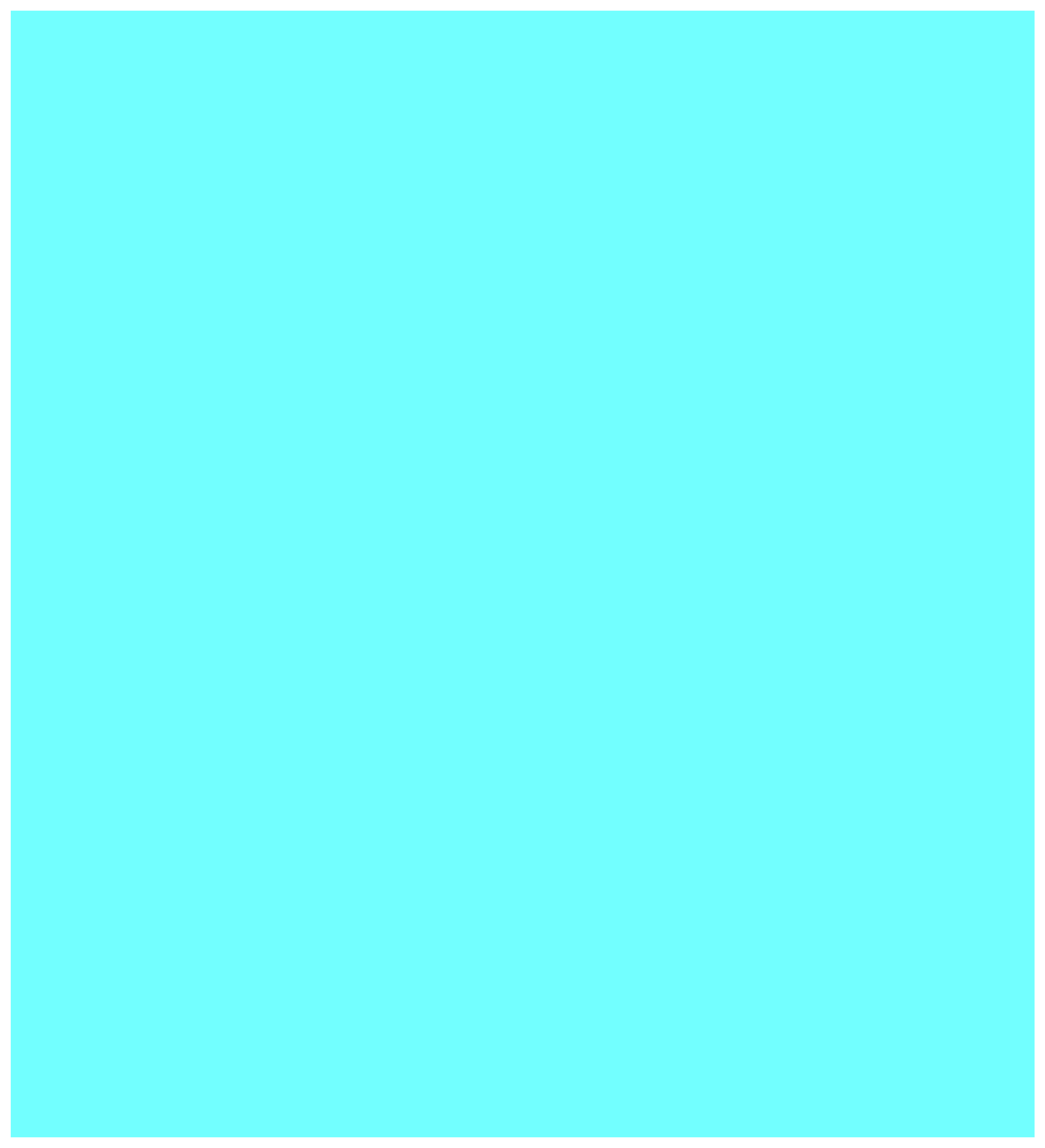# color_loop()

The `color_loop()` function described below will iterate over a vector of color names and display each color. The function creates an empty `ggplot2` plot that has its background sequentially filled with the given colors. Optionally, the name or hex value for the color will be shown in the plot as well. The delay between each color and the number of iterations are also parameters.

``````#' Color loop
#'
#' @param colors Vector of colors over which to loop
#' @param loop Number of iterations; default is `10`
#' @param delay Delay in seconds between each color; default is `0.5`
#' @param label Logical to show the hex value or name for the color; default is `FALSE`
#' @param label_color Character vector to specify the color of the label text if applicable; default is `'black'`
#' @param boomerang Logical as to whether or not to reverse loop in each iteration; default is `FALSE`

color_loop <- function(colors =  c("red", "green", "blue", "yellow", "violet"),
loop = 10,
delay = 0.5,
label = FALSE,
label_color = "black",
boomerang = FALSE) {

clock <- 1
runtime <- loop * (length(colors) * delay)
message(sprintf("EXPECTED RUNTIME: %#.1f SECONDS", round(runtime, 2)))
while(clock < loop) {

for(color in seq_along(colors)) {

if(label) {
p <-
ggplot2::ggplot() +
ggplot2::geom_text(ggplot2::aes(x = 1,
y = 1,
label = colors[color]),
size = 40,
color = label_color) +
ggplot2::theme_void() +
ggplot2::theme(panel.background = ggplot2::element_rect(fill = colors[color]))
} else {
p <-
ggplot2::ggplot() +
ggplot2::theme(panel.background = ggplot2::element_rect(fill = colors[color]))
}
print(p)
Sys.sleep(delay)
}

if(boomerang) {
colors <- rev(colors)
}
clock <- clock + 1

}

}``````

The examples below show the function in action with different color scales.

Note that the images in this post are .gif files captured for demonstration purposes and will continuously loop. If used interactively, the function will iterate a fixed number of times as specified by “loop” argument (default 10).

``````rgbyv <- c("red", "green", "blue", "yellow", "violet")
color_loop(colors = rgbyv, label = TRUE, delay = 1)````````````cms <- cm.colors(20)
color_loop(colors = cms, delay = 0.1, boomerang = TRUE)``````